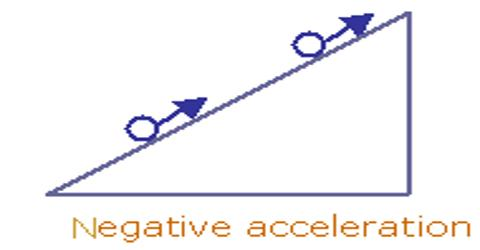Physics

# Retardation: DefinitionRetardation is a negative acceleration or deceleration. The velocity of a body may increase or decrease. Positive acceleration increases the speed of a moving object so the object moves faster and faster. Negative acceleration or deceleration has the opposite effect and it reduces the speed of a moving object. When the velocity of a body increase, the acceleration is said to be positive and when the velocity of a body decrease, the acceleration is said to be negative (Retardation). If the velocity of a body is decreasing with respect to time, the acceleration is said to be negative. Retardation is the rate of decrease in velocity.

When we say retardation then that means that the director of change is opposite to the current velocity so it will decrease. Retardation eats the current velocity until it becomes 0 and then the body will start moving in the opposite direction.

It is the rate at which an object slows down. Retardation is the last velocity minus the initial velocity, with a negative sign in the consequence because the velocity is dropping. The formula for acceleration can be used, recognizing that the final result must have a negative sign. And if time is also given then retardation = (final velocity – initial velocity) / time.

Velocity is a measure of how quickly an object moves. So, the velocity is the change in the position of an object, divided by the time.

Velocity= (final position- initial position)/time

Or, Velocity= (change in position)/ time

The SI unit of retardation is the same as that of acceleration, which is meter per second squared (m/s2)

Example:

If the driver pressed on the brake instead of accelerator then the motion of the motor-cycle becomes slower. Its velocities may decrease to 5 m/s per second. The rate of decrease in velocity of the motor-cycle per unit time is negative acceleration or retardation.

Another example – a train is said to be retarded when it slows down on reaching a station, i.e. the velocity decreases. Retardation is acceleration with a negative sign. Or the negative value of acceleration shows that the velocity of a body is decreasing.

Some common examples of retardation motion are: Velocity of the vehicle decreases when the brake is applied, Landing of Aeroplane, When a fan is switched off, When a train reaching a station, When a roller costers are about to stop, Drawing water from well, pumping a hand pump, etc.